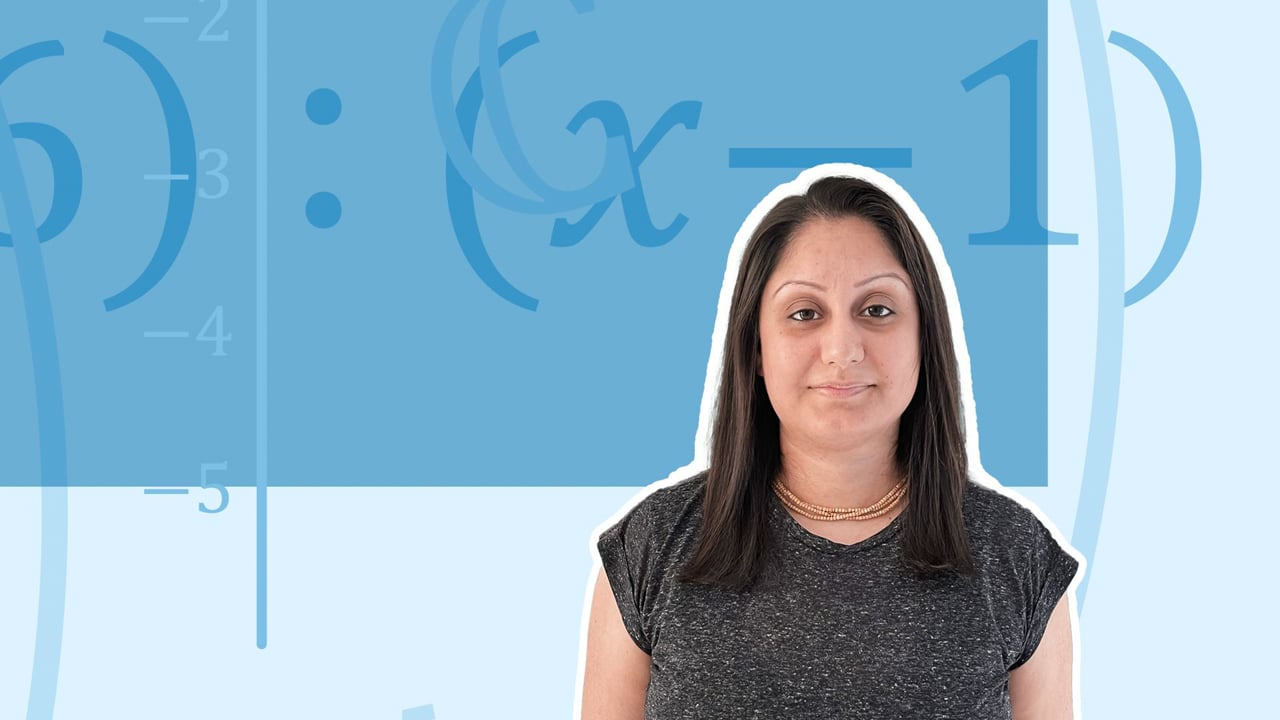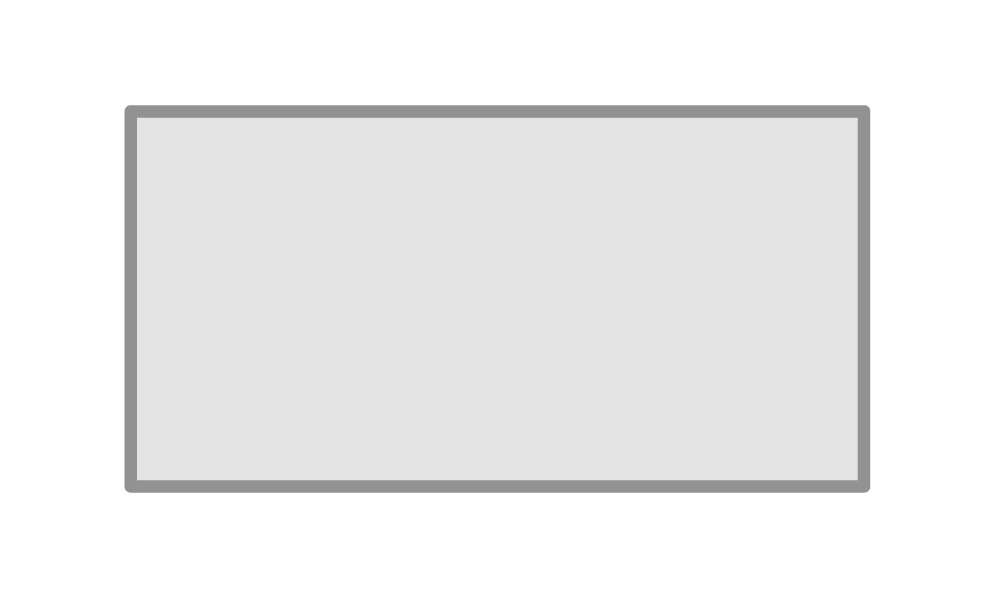Chapter overviewMaths

Exam board

AQA

Number

Algebra

Graphs

Ratio proportion and rates of change

Shapes and area

Angles and geometry

Trigonometry

Probability

Statistics

Maths

# Writing formulae and equations from diagrams0%

Summary

# Writing formulae and equations from diagrams

## In a nutshell

You should be able to form expressions or formulae from a diagram and information given in a question. Start by giving the unknown quantities a letter name, e.g. $x$. Then use the information in the question to create your expression or formula. For 2D shapes, you may have to form an equation for perimeter or area, and for 3D shapes you may have to form an equation for volume or surface area.

Note: Remember to substitute into the formula to check that is works.

## Make a formula

To make a formula, read the information given, give the unknown quantity a letter name, and use your knowledge of algebra to give the formula. Label the diagram to help.

##### Example 1

A rectangle has length $4cm$ more than the width. Write formulae for the area and perimeter of the rectangle.

First draw a labelled diagram, label the unknown width $x$, if the length is $4$ more than the width, label this $x+4$.​

 ​$x+4$​​ ​$x$​​\begin {aligned} Area &= width \times length \\ A&= x (x+4) \\A&= \underline{x^2 + 4x}\end {aligned}

\begin {aligned}Perimeter &= x + x +x+4 + x+4 \\P &= \underline{4x+4} \\\end {aligned}​​

##### Example 2

A sector has radius $r$, and an angle of $120\degree$

Write an equation for the perimeter of the shape and find the perimeter when $r = 5$

​​

The arc length is given by

$Arc \space length = \frac {120} {360} \times \pi r^2$

Therefore, the total perimeter is

\begin {aligned} P &= \frac {120} {360} \times \pi r^2 + r + r \\P &=\underline{ \frac 1 3 \pi r^2 +2r} \end {aligned}​​

Now, use the formula with $r=5$

\begin {aligned}P &= \frac 1 3 \pi \times 5^2 + 2 \times 5 \\P &= \underline{36.2}\end {aligned}​​

\begin {aligned}A&= width \times length \\A&= x(x+4) \\A&=x^2+4x\end {align